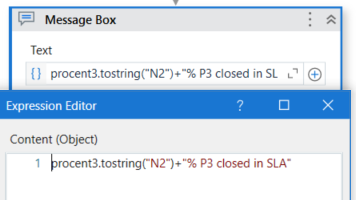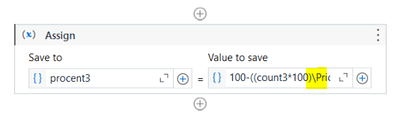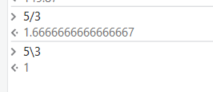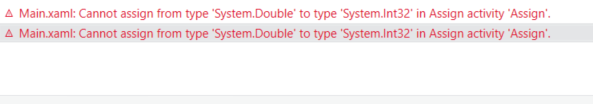# How to obtain the result of a formula with 2 decimals

Hi all,

I have this situation: My robot has to do a math formula pretty easy and to return a number with 2 decimals and I tried everything I could find. This is how it looks so far.
The formula is 100-((count3*100)\Prio3) and it should return a number like this xx.xx . The vars count and Prio3 are integers, and they will always be integers.

The output is a double variable at the moment and the message will be rounded up.I tried changing the procent3 var into decimal and the same result.
I don’t need to Round up the result. I need it as it is but within 2 decimals.
P.S.: The vars count and Prio3 are obtain after the robot searches within an excel sheet how many rows fulfils a condition.

Any ideas?

Hello @Andreea_Nistor
Check the Variable type of Procenr3 is a System.Double, If it is in Integer, it will round off the value.

Hi,

I think the above expression returns integer.

The following expression might be what you expect.

`````` 100-((count3*100)/Prio3)
``````

Regards,

kindly note:\ is so called Integer division

well I didn’t know that, but if i use “/” instead of "" it give me an errorit’s a double. but I am thinking that might be something wrong with the formula…

Hi,

In my environment,`/` works as the following.

Can you check type of variable?

or

Try to use double literal explicitly, as the following.

``````  100R-((count3*100R)/Prio3)
``````

Regards,

Did some other modifications and this worked. Ty!

This topic was automatically closed 3 days after the last reply. New replies are no longer allowed.